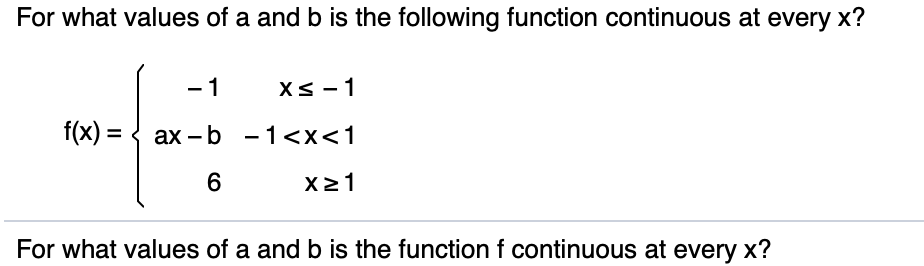# For what values of a and b is the following function continuous at every x?-1xs - 1f(x) < ax b -1

Questionhelp_outlineImage TranscriptioncloseFor what values of a and b is the following function continuous at every x? -1 xs - 1 f(x) < ax b -1
check_circleExpert Solution
Step 1

At x=-1 , left hand limit=-1

Right hand limit= a(-1)-b= -a-b

The function is continuous so left hand limit = right hand limit

-1 = -a - b

Or, a+b= 1

Step 2

At x=1 , left hand limit= a(1)-b=a-b

Right hand limit= 6

The function is continuous so left hand limit = right hand limit at x=1

a - b=6

Step 3

a+b=1

a-b=6

From these two equations we have to find a and b.

If...

### Want to see the full answer?

See Solution

#### Want to see this answer and more?

Solutions are written by subject experts who are available 24/7. Questions are typically answered within 1 hour*

See Solution
*Response times may vary by subject and question
Tagged in

### Other